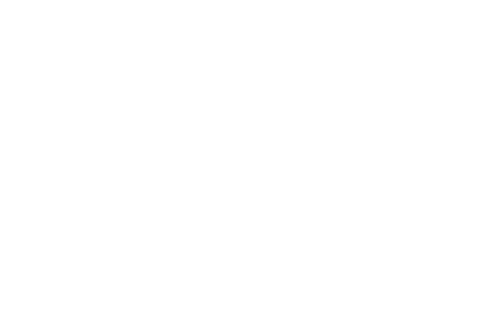# Caroline Bentley## Mrs. Kraatz

#### 6th GradeMath, Science, and Social StudiesBentleyakraatz@nlsd122.org

Chapter 13 - Matter

Lesson 1 What is Matter?

Lesson 2 How can Matter Change?

Chapter 14 - Building Blocks of Matter

Lesson 1: How did we learn about atoms?

Lesson 2: How are elements grouped?

Lesson 3: What are compounds and mixtures?

Chapter 15- Forces & Motion

Lesson 1: What happens when forces act on an object?

Lesson 2: How does gravity affect objects?

Lesson 3: How can you describe motion?

Lesson 4: What are the laws of motion?

Chapter 2 - Cells

Lesson 1: What is a cell?

Lesson 2: What are the functions of organelles?

Lesson 3: How do cells grow and divide?

Chapter 3 - Reproduction